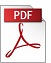### “A Boundary Element Solution to the Problem of Interacting AC Fields in Parallel Conductors”

Authors: Einar M. Rønquist and Terje Sira,
Affiliation: Institute for Energy Technology (IFE)
Reference: 1984, Vol 5, No 2, pp. 103-111.

Keywords: (No keywords)

Abstract: The ac fields in electrically insulated conductors will interact through the surrounding electromagnetic fields. The pertinent field equations reduce to the Helmholtz equation inside each conductor (interior problem), and to the Laplace equation outside the conductors (exterior problem). These equations are transformed to integral equations, with the magnetic vector potential and its normal derivative on the boundaries as unknowns. The integral equations are then approximated by sets of algebraic equations. The interior problem involves only unknowns on the boundary of each conductor, while the exterior problem couples unknowns from several conductors. The interior and the exterior problem are coupled through the field continuity conditions. The full set of equations is solved by standard Gaussian elimination. We also show how the total current and the dissipated power within each conductor can be expressed as boundary integrals. Finally, computational results for a sample problem are compared with a finite difference solution.PDF (1785 Kb)        DOI: 10.4173/mic.1984.2.2

References:
 ABRAMOWITZ, N1., STEGUN, I.A. (1965). Handbook of Mathematical Functions, Dover.
 ANDERSEN, O.W. (1977). Finite element solution of skin effect and eddy current problems, IEEE paper, A 77616-6.
 CARPENTER, C. J. (1977). Comparation of alternative formulations of 3-dimensional magnetic-field and eddy-current problems at power frequencies, Proc. IEE, 124, 66-74.
 CHARI, M.V.K. (1973). Finite element solution of the eddy current problem in magnetic structures, IEEE paper, T 73 320-9.
 EKRANN, S., SIRA, T. (1982). A model for solving the Maxwell quasi-stationary equations in a three phase electric furnace, Modeling, Identification and Control, 3, 231-242 doi:10.4173/mic.1982.4.4
 SALON, S.J., SCHNEIDER, J.M., UDA, S. (1981). Boundary element solutions to the eddy current problem, Proc. of the 3rd international seminar on boundary element methods.Springer Verlag, pp. 14-25.

BibTeX:
@article{MIC-1984-2-2,
title={{A Boundary Element Solution to the Problem of Interacting AC Fields in Parallel Conductors}},
author={Rønquist, Einar M. and Sira, Terje},
journal={Modeling, Identification and Control},
volume={5},
number={2},
pages={103--111},
year={1984},
doi={10.4173/mic.1984.2.2},
publisher={Norwegian Society of Automatic Control}
};